Benjamin S. Valle

email: calmansys@yahoo.com

Pressure Measurements

The measurement of pressure is considered the basic process variable
in that it is utilized for measurement of flow (difference of two pressures),
level (head or back pressure), and even temperature
(fluid pressure in a filled thermal system)
Pressure definition
Pressure is the action of one force against another over, a surface.
The pressure P of a force F :distributed over an area A is defined as
P = F/A
Pressure and Area:
For practical purposes, Pressure can be varied considerably for
any fixed available Force by changing the surface Area. This is
the underlying concept of Hydraulic Systems.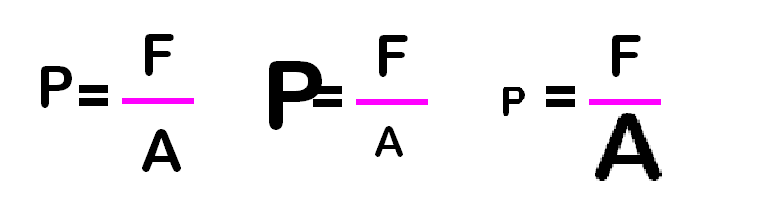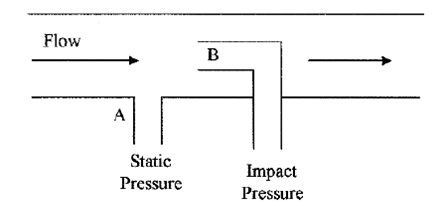Static, dynamic, and impact pressure
Static pressure is the pressure of fluids or gases that are
stationary or not in motion.

Dynamic pressureis the pressure exerted by a fluid or gas when
it impacts on a surface or an object due to its motion or flow.
In the diagram, dynamic pressure is (B-A).
Impact pressure (total pressure) is the sum of the static and
dynamic pressures on a surface or object.
Point B in the diagram depict the impact pressure.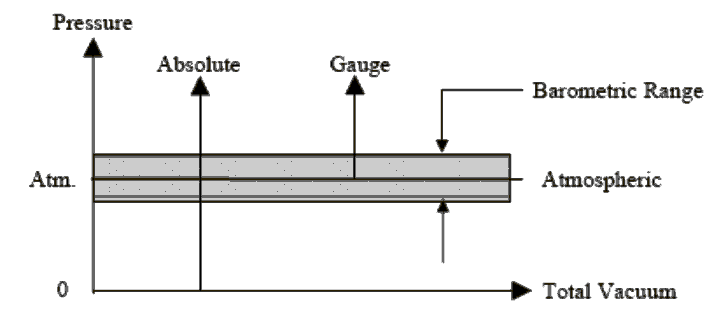Reference or starting point of different Pressure Types

Absolute pressure

The pressure is referenced to zero absolute pressure and has
units of psia. Absolute pressure can only have a positive value

Gauge pressure

The pressure is referenced to atmospheric pressure and by
convention is measured in the positive direction, i.e. 7 psig
Compound pressure
The pressure is referenced to atmospheric pressure and Has
both a positive and negative component, i.e. -0.5" H2O To +0.5" H2O
Vacuum pressure
The pressure is referenced to atmospheric pressure and by
convention is measured in the negative direction, i.e. -50 mm Hg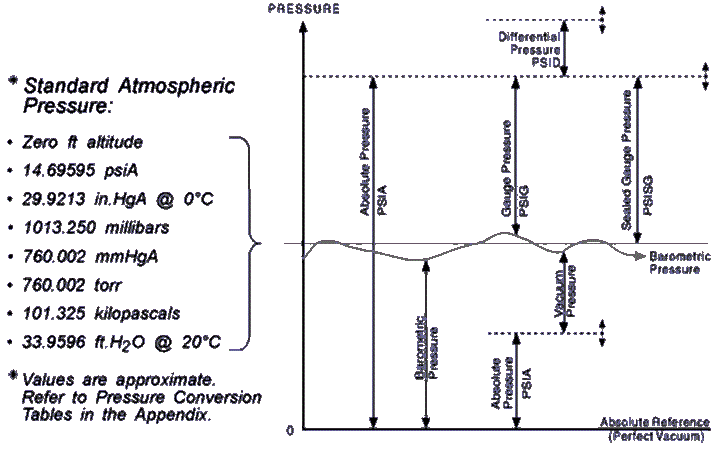Standard Atmospheric Pressure
Pa = Absolute Pressure
Pg = Gauge Pressure
Patm = Atmospheric Pressure
Pa = Pg + Patm (when Pg > Patm)
Pa = Pg - Patm (when Pg < Patm)
Example
Problem: If a pressure instrument has a reading of 30 psig,
find the absolute pressure if the local barometric reading is 14.6 psi
Solution: Since Pg > Patm
Pa = Pg + Patm
Pa = 30 psi + 14.6 psi
Pa = 44.6 psia
Problem: Find the absolute pressure if a vacuum gauge reads
11.5 psig and the atmospheric pressure is 14.6 psia.
Solution: When dealing with pressure below atmospheric pressure
Pa = Pg – Patm
Pa = 11.5 psi – 14.6 psi
Pa = 3.1 psia

Definition of Pressure Terms
Differential Pressure
The difference in magnitude between two related pressures
Hydrostatic Pressure
The pressure below a liquid surface exerted by the liquid above
Line Pressure (Static Pressure)
Force per unit area exerted on a surface by a fluid
flowing parallel to a pipe wall
Vacuum
Pressure below atmospheric
Pressure Measurement Units
A number of measurement units are used for pressure.
They are as follows:
1. Pounds per square foot (psf) or pounds per square inch (psi)
2. Atmospheres (atm)
3. Pascals (N/m2) or kilopascal (1000Pa)*
4. Torr = 1 mm mercury
5. Bar (1.013 atm) = 100 kPa
6. 14.696 lbf/in2 equals 33.9 feet of H2O
7. 14.696 lbf/in2 equals 29.921 inches of of Hg

Atmospheres (atm)
Pascals (N/m2) or kilopascal (1000Pa)
Torr = 1 mm mercury

Bar (1.013 atm) = 100 kPa

14.696lbf/in2 equals 33.9 feet of H2O
14.696lbf/in2 equals 29.921 inches of of Hg

Atmospheres

In 1954 the 10th Conférence Générale des Poids et Mesures (CGPM)
adopted standard atmosphere for general use and affirmed its definition
of being precisely equal to 1,013,250 dynes per square centimeter (101,325 Pa).
This value was intended to represent the mean atmospheric pressure
at mean sea level at the latitude of Paris, France, and as a practical matter,
truly reflects the mean sea level pressure for many of the
industrialized nations (those with latitudes similar to Paris).
Pascals
The SI unit for pressure is the pascal (Pa),
equal to one newton per square meter.
A Newton (N), the SI unit of force,
is equal to the force required to accelerate 1 kilogram
of mass at a rate of 1 meter per second squared.
Thus a Pascal is equal to the pressure of 1 newton
over a surface area of 1 square meter
Torr
The torr (symbol: torr) is a non-SI unit of pressure defined
as 1/760 of an Atmosphere. It was named after
Evangelista Torricelli, an Italian physicist and mathematician
who discovered the principle of the barometer in 1644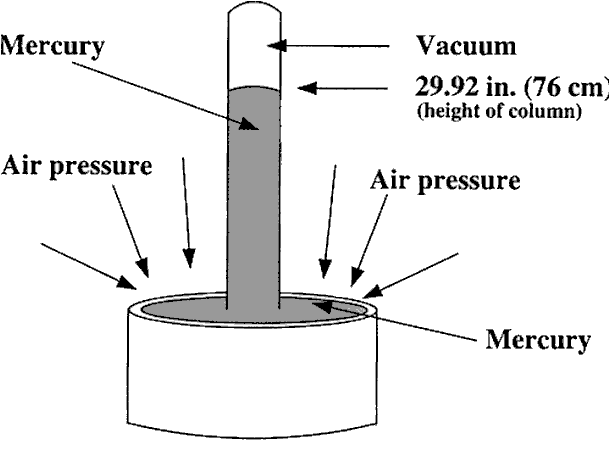Torricelli's mercury barometer
The weight of the mercury in the tube is balanced by the
force of the air on the mercury in the dish
Bar
The word bar has its origin in the Greek word βÎ¬ρο (baros), meaning weight.
Its official symbol is "bar" the earlier "b" is now deprecated, but still often see
especially in "mb" rather than the proper "mbar" for millibars
The bar and millibar were introduced by Sir Napier Shaw in 1909
Example
What pressure in pascals corresponds to 15 psi?
p = 15 psi (6.895 kPa/psi) = 102.9 kPa

Class Exercise
What pressure, in psi, would be indicated by an absolute pressure gauge
that is lying on a workbench not connected to anything?
Pressure Units
As previously noted, pressure is force per unit area and historically
a great variety of units have been used, depending on their
suitability for the application.
For example, blood pressure is usually measured in mmHg.
because mercury manometers were used originally.
Atmospheric pressure is usually expressed in mmHg for the same reason.

Pressure Units
The following conversion factors should help in dealing with the various units

1psi = 51.714 mmHg
= 2.0359 in.Hg
= 27.680 in.H2O
= 6.8946 kPa
1 bar = 14.504 psi
1 atm = 14.696 psi

Example
Convert 200 mmHg to psi
200mmHg x 1 psi/51.714 mmHg = 3.867 psi
Class exercises
If a cubic foot of water weighs 62.4 pounds, it exerts a pressure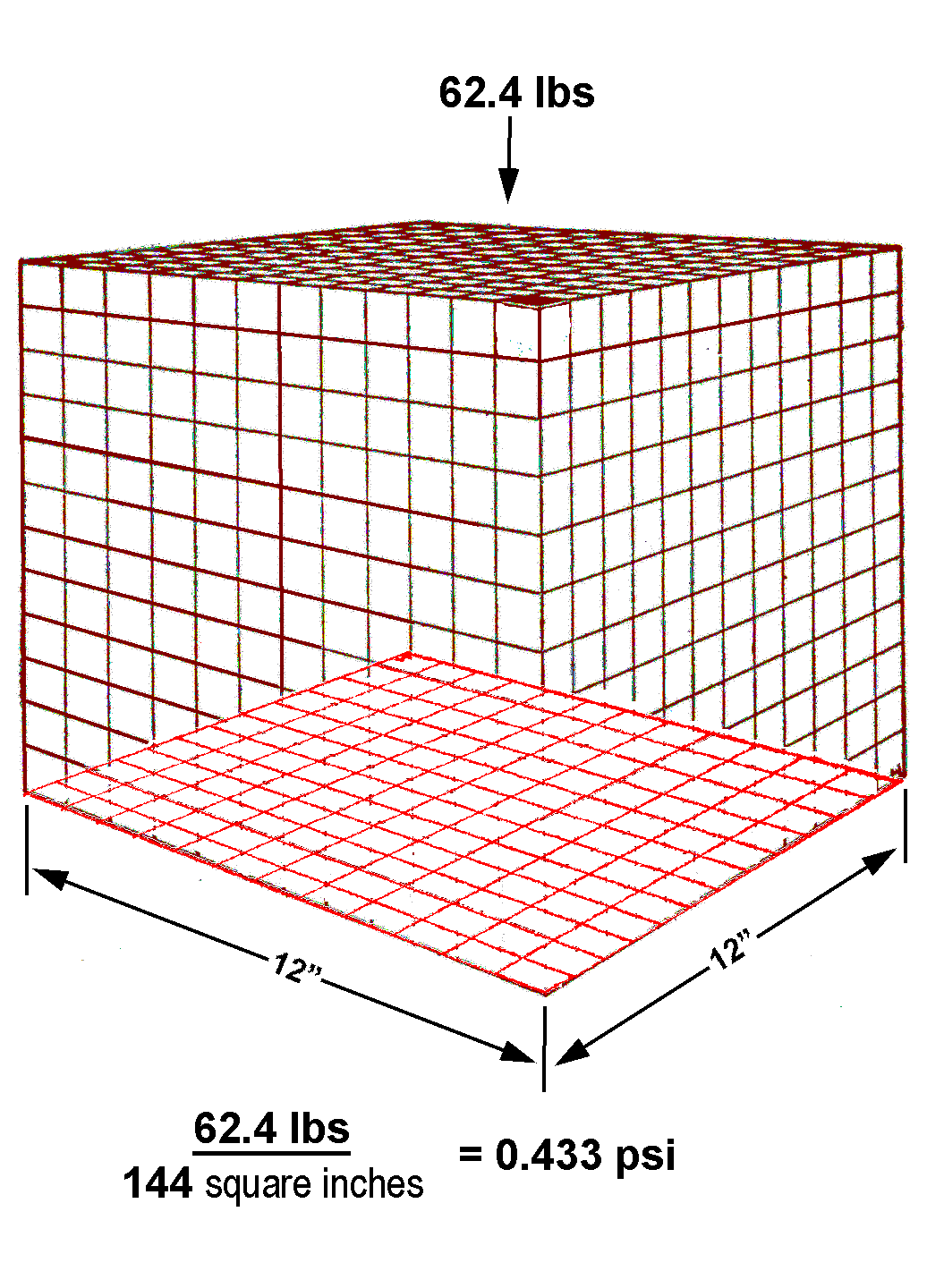of how many pounds per square inch (psi)?
And what is approximately the pressure, in psi, at the bottom of
a 20-foot tank filled with a fluid that has a specific gravity of 1.5

Hint
Remember that pressure is force (weight) per unit area and that
Specific gravity (SG) is the density (mass/volume) of a substance
with reference to the density of water. Also remember that
a substance, which has an SG of 1.5, as in this problem,
has 1.5 times the mass of water
Solution:
Imagine a cubic foot of water. It's 12 inches wide,
12 inches back, and 12 inches high.
Because the base of this cube of water is 12 by 12,
we know that the base is 144 square inches.

The question asks how many pounds per square inch does
the box exert. Put 62.4 pounds in the numerator and 144
square inches in the denominator, divide, and you get 0.433 psi

Multiply it by 20, we get the psi of the 20-foot-high column
Now we multiply that by 1.5 because the substance in this
problem has 1.5 times the mass of water (0.433 x 20 x 1.5 = 12.99)

Hydrostatic pressure is the pressure which rises above a
certain level in a liquid owing to the weight of the liquid mass
ps =
h * ρ * g
ps = hydrostatic pressure (gravitational pressure) [Pa]
h = level of the column of liquid [m]

ρ = density of the liquid [kg/m3]
g = acceleration due to gravity [m/s2]
In accordance with the SI international system of units, hydrostatic pressure
is given in both Pascal and bar. The level of the column of liquid
is given the unit “metre”, the density of the liquid “kilograms per cubic metre”
and the acceleration due to gravity “metres per second squared".
The hydrostatic pressure, or simply “pressure” as it is known for short,
does not depend on the type of vessel used. It is purely dependent
on the height and density of the column of liquid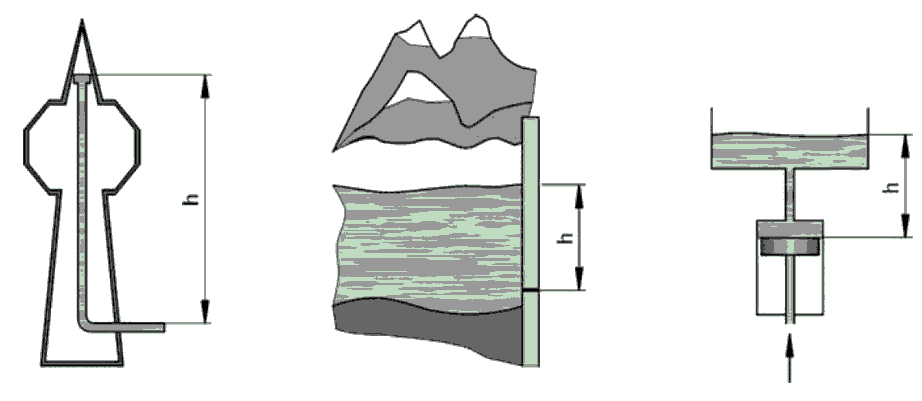Column = 300m Reservoir = 15m Elevated Tank = 5m
Which of the three structures will give the highest pressure reading at the base?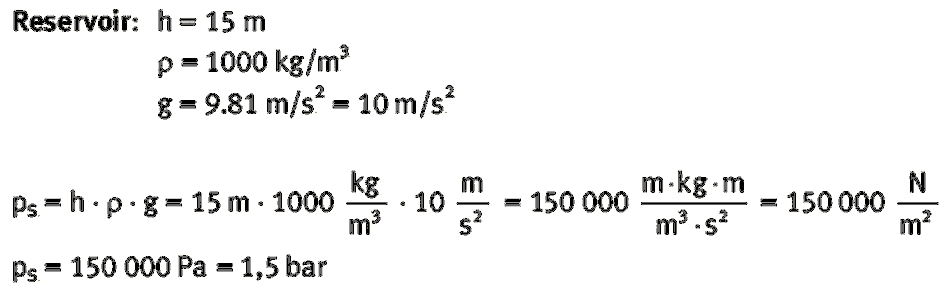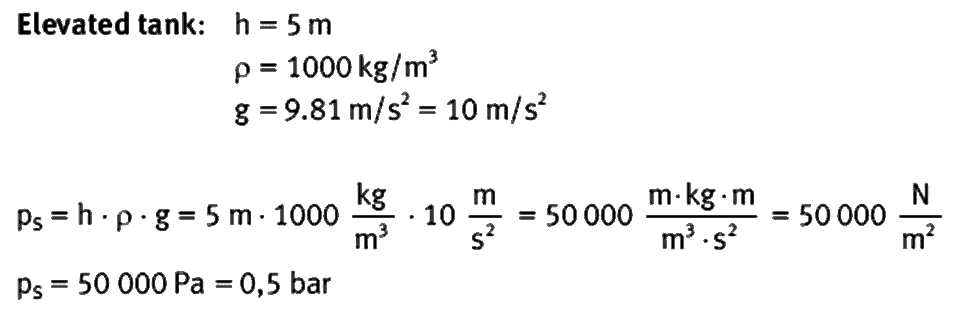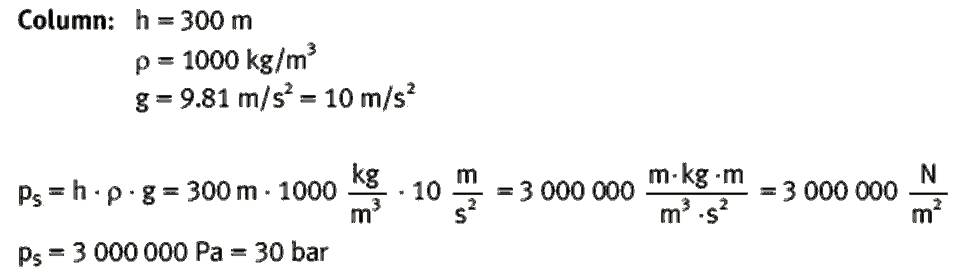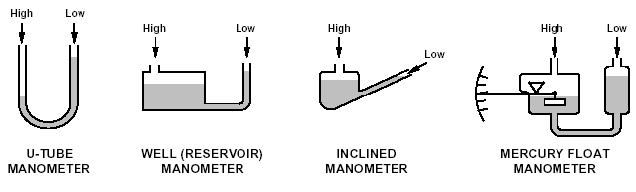Manometer basics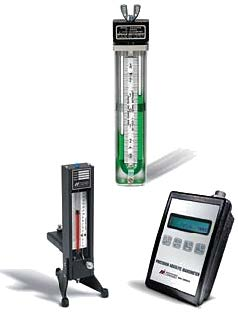Characterized by its inherent accuracy and simplicity of operation. It’s the U-tube manometer, which is a U-shaped

glass tube partially filled with liquid.
This manometer has no moving parts and requires no calibration.
Manometer measurements are functions of gravity and the liquid’s density, both physical properties that make the U-tube manometer a NIST standard for accuracy.

With both legs of a U-tube manometer open
to the atmosphere or subjected to the same pressure,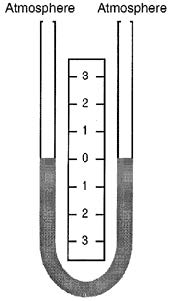the liquid maintains the same level in each leg,
establishing a zero reference.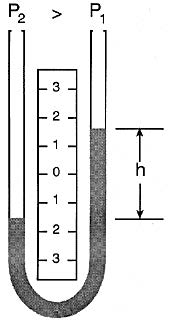With a greater pressure applied to the left side of a U-tube manometer, the liquid lowers in the left leg and rises in the right leg.

The liquid moves until the unit weight of the liquid, as indicated by h, exactly balances the pressure.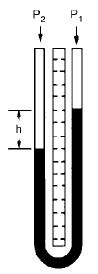When the liquid in the tube is mercury, for example, the indicated pressure his usually expressed in inches of mercury. To convert to pounds per square inch, P2 = dh
Where
P2 = pressure, psig
d= density, lb/in3
h= height, inches
For mercury, the density is 0.490 lb/in3 at 60OF (15.6OC),
and the conversion of inches of mercury to
pounds per square inch becomes
P2 = 0.490h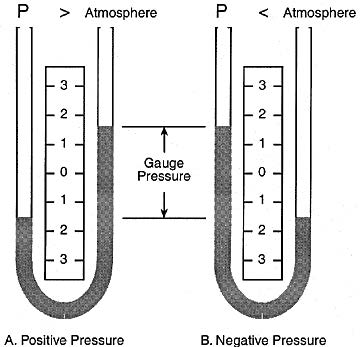Gauge pressure is a measurement relative to atmospheric pressure and it varies with the barometric reading.
A gauge pressure measurement is positive when the unknown pressure exceeds atmospheric pressure (A),
and is negative when the unknown pressure is less than atmospheric pressure (B).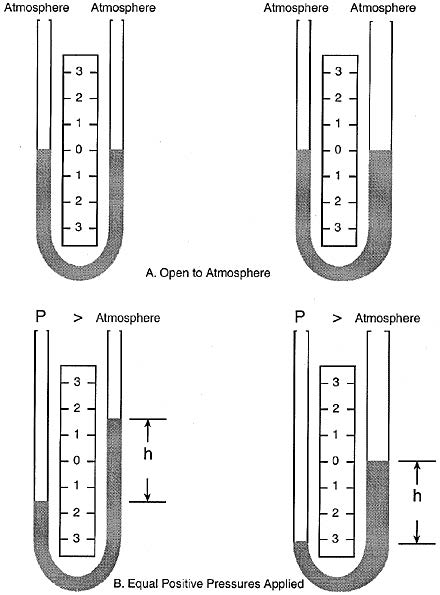Variations on the U-Tube
Manometer
The pressure reading is always the difference between fluid heights, regardless of the tube sizes.
With both manometer legs open to the atmosphere, the fluid levels are the same (A).
With an equal positive pressure applied to one leg of each manometer, the fluid levels differ, but the distance between the fluid heights is the same (B).

Reservoir (Well) Manometer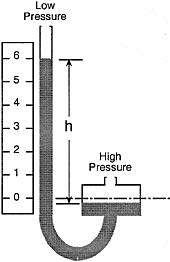In a well-type manometer,the cross-sectional area of one leg (the well) is much larger than the other leg. When pressure is applied to the well, the fluid lowers only slightly compared to the fluid rise in the other leg.
One leg of the manometer is increased many times in area to that of the other the volume of fluid displaced will represent very little change of height in the smaller area leg. This condition results in an ideal arrangement whereby it is necessary to read only one convenient scale adjacent to a single indicating tube rather than two in the U-type.
The larger area leg is called the well. The true pressure still follows the principles previously outlined andis measured by the difference
between the fluid surfaces.
It is apparent that there must be some drop in the well level. This is readily compensated for by spacing the scale graduations in the exact amount required to reflect and correct for this "well drop".

Inclined-tube Manometer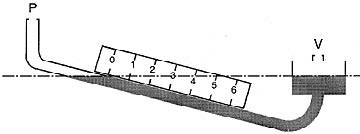With an inclined indicating tube, 1 in. of a vertical rise is stretched over several inches of scale length. The inclined-tube manometer has better sensitivity and resolution for low pressures.
Low pressure and low differentials are better handled with an inclined-tube manometer, where 1 in. of vertical liquid height can be stretched to 12 in. of scale length.

Exercise
When reading a mercury manometer, the center of the meniscus should be
A. Higher than the outer edge
B. Lower than the outer edge
C. Same as the outer edge
D. Level to the outer edge
Exercise Hint

The meniscus is the characteristic upward or downward curving shape
of a liquid in a glass or tube such as a manometer.
A meniscus happens because there are characteristic surface tensions,
and adhesive and cohesive forces at work in liquids.

If water is in a tube, for example, the adhesive forces between
the glass and the water is stronger than the cohesive forces among
the water molecules themselves
Thus,one sees a concave or downward curving meniscus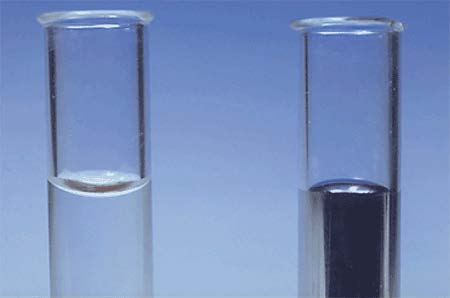With mercury, the cohesive forces are much greater
than the adhesive forces toward the glass wall,
and it has an upward curving or convex meniscus.

Pressure Sensing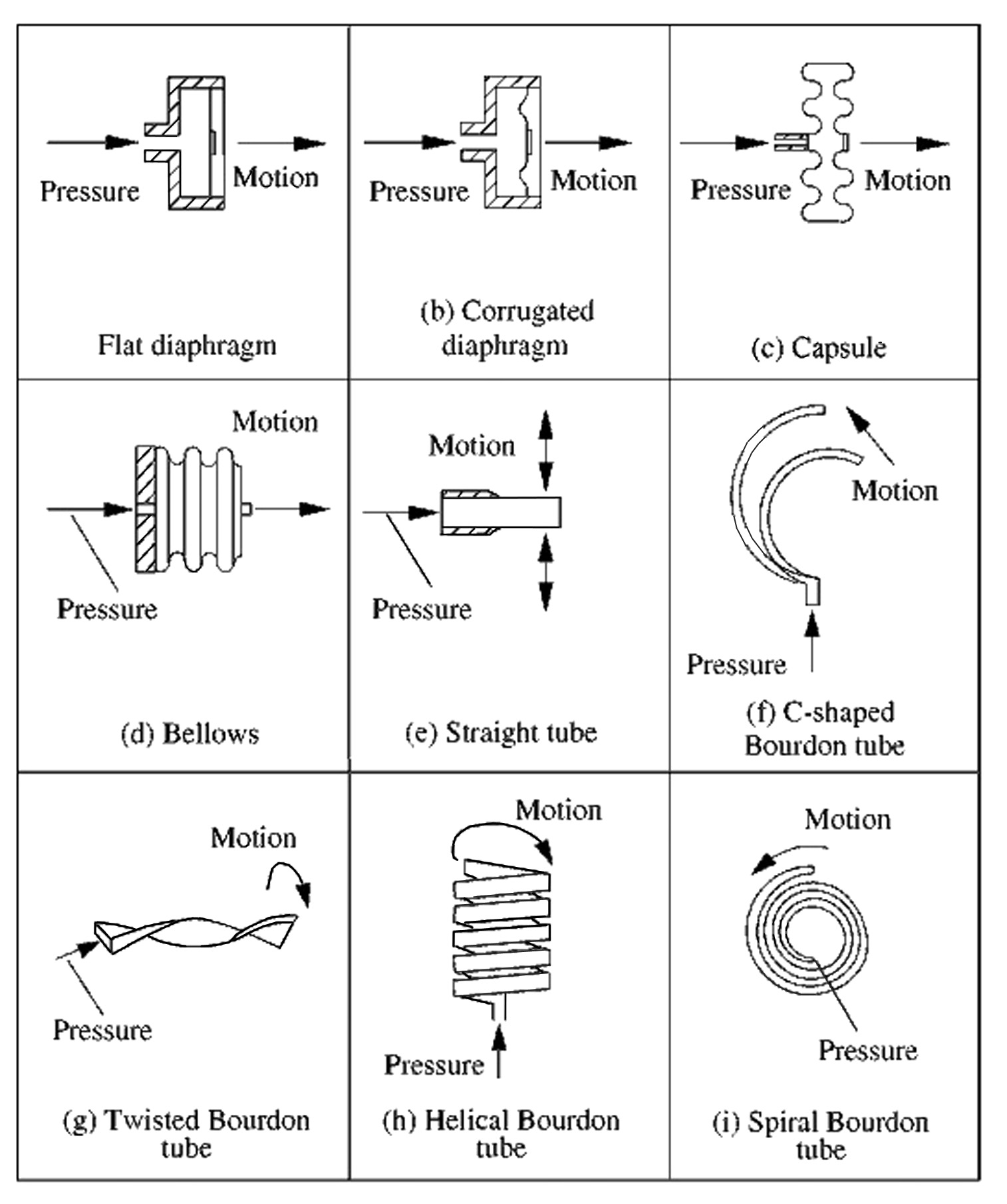Pressure is sensed by mechanical elements that are designed and constructed
to deflect when pressure is applied.

This is the basic mechanism converting pressure to physical movement.
Next, this movement is converted to an electrical signal for remote indication
Finally, signal conditioning may be needed,
depending on the type of sensor and the application.
The basic pressure sensing element can be configured as
a C-shaped Bourdon tube (F); a helical Bourdon tube (H); flat
diaphragm (A); a convoluted diaphragm (B); a capsule (C); or bellows (D).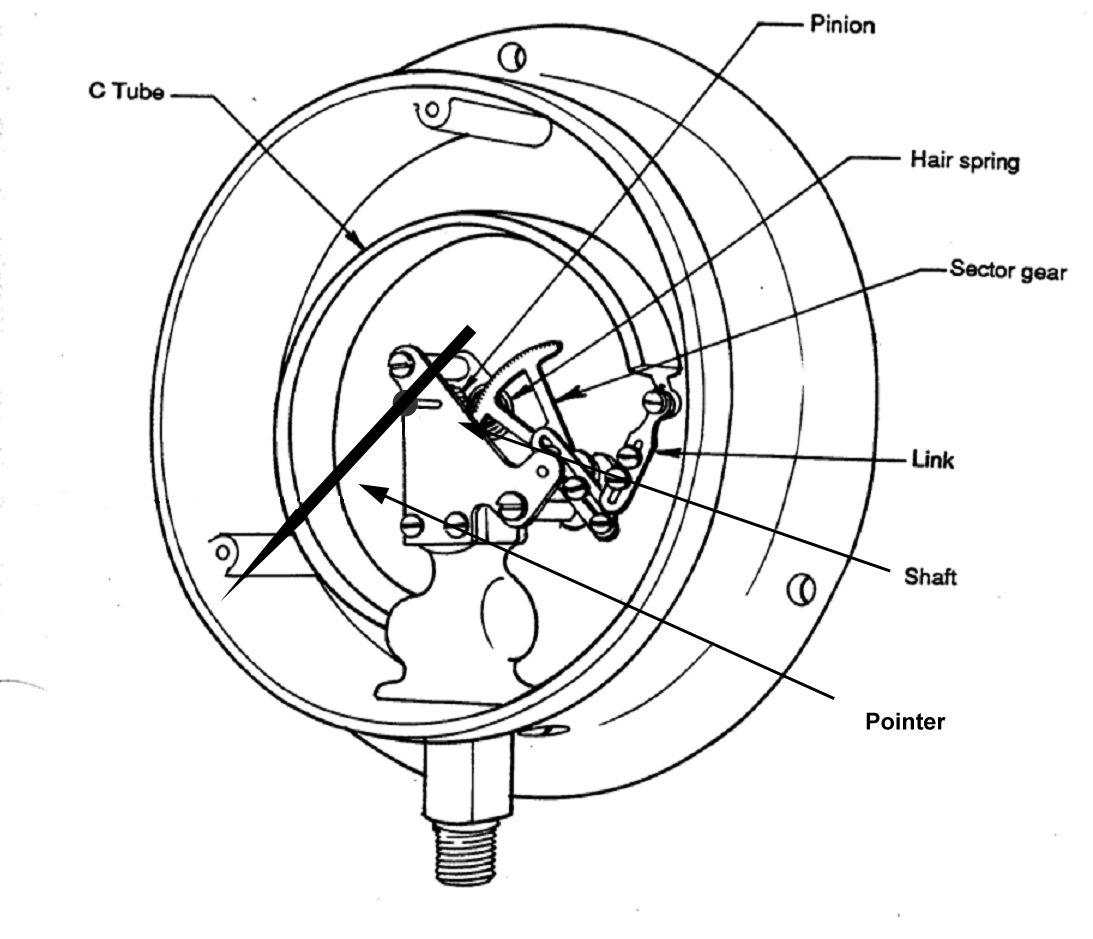C type Bourdon Tube Pressure Gauge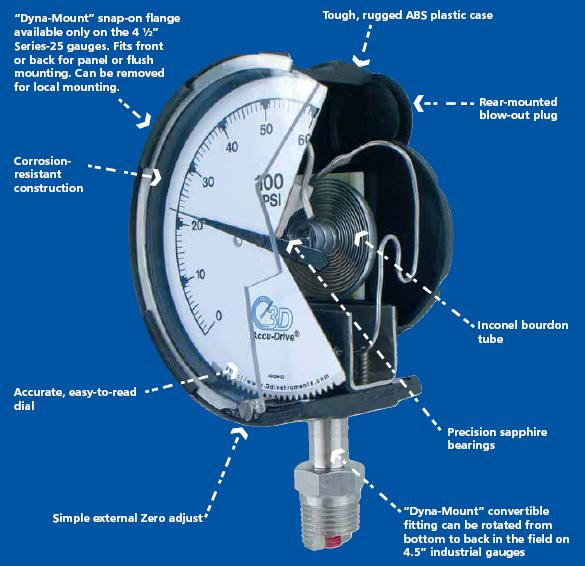In “C” type Bourdon tube, a section of tubing that is closed
at one end is partially flattened and coiled.
When a pressure is applied to the open end, the tube uncoils. This movement provides a displacement that is proportional to the applied pressure.
The tube is mechanically linked to a pointer on a pressure dial to give a calibrated reading.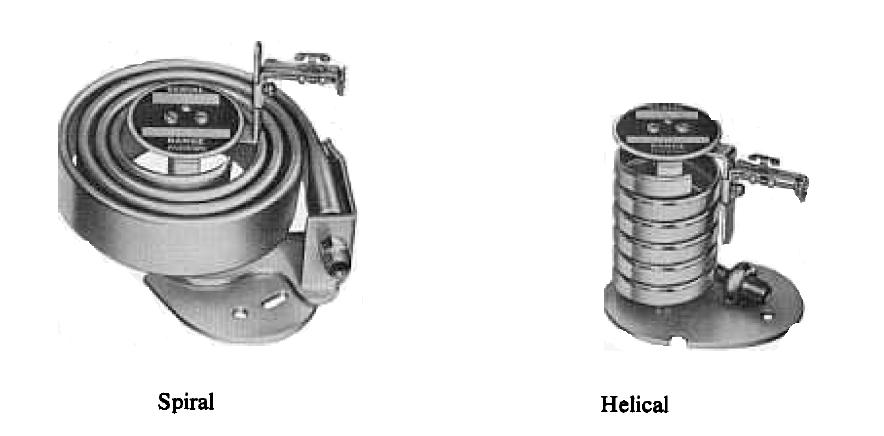Helical and Spiral Bourdon Tubes Spiral Bourdon Tube Gauge
Bourdon Helix type are characterized by the following:
High over range (100%)
High pressure approximately 20 coils withstand up to 80,000 psi
Low pressure approximately 3 coils withstand up to 30 psi
Accuracy from 0.25% to 5% of span
Quite often used in filled thermal systems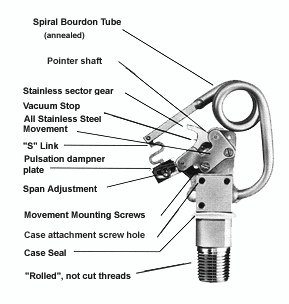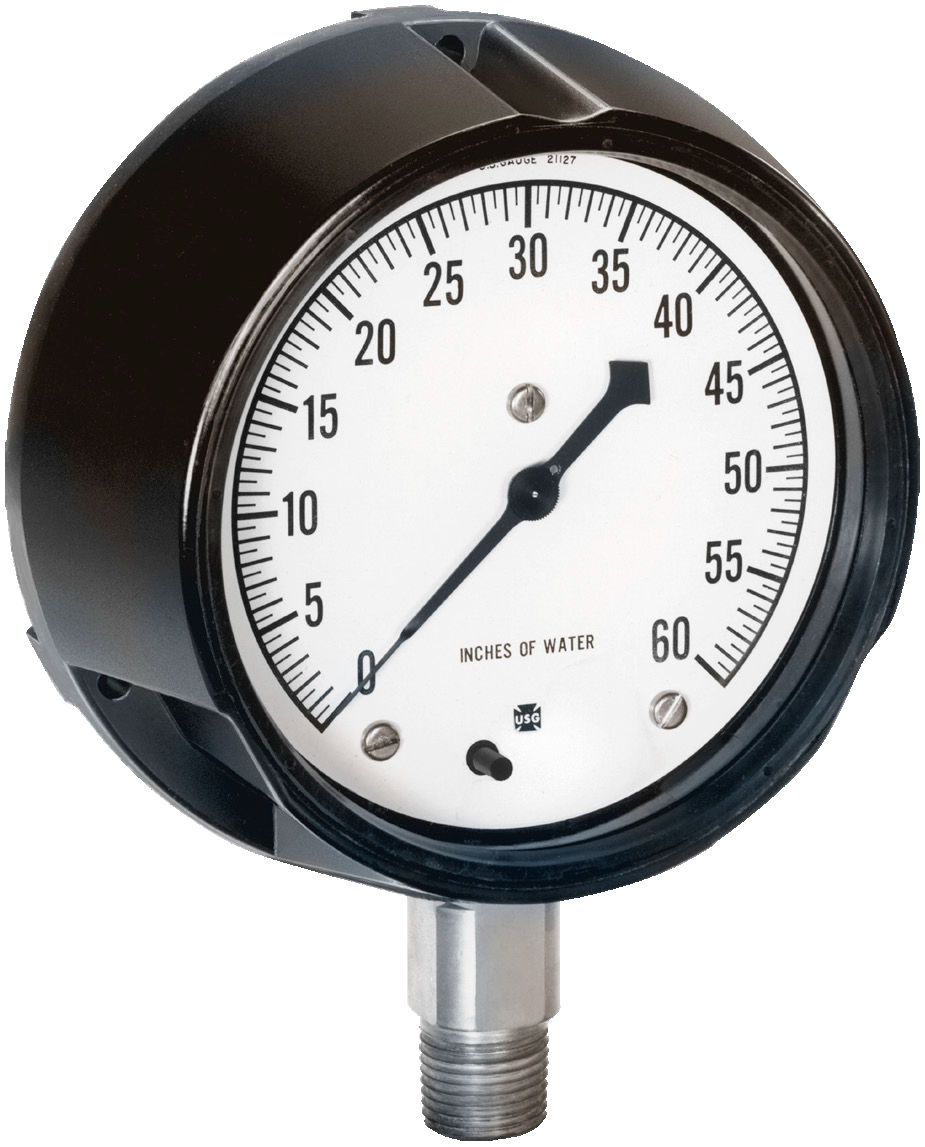Helical BourdonTube Gauge

Low Range Diaphragm Type Pressure Gauge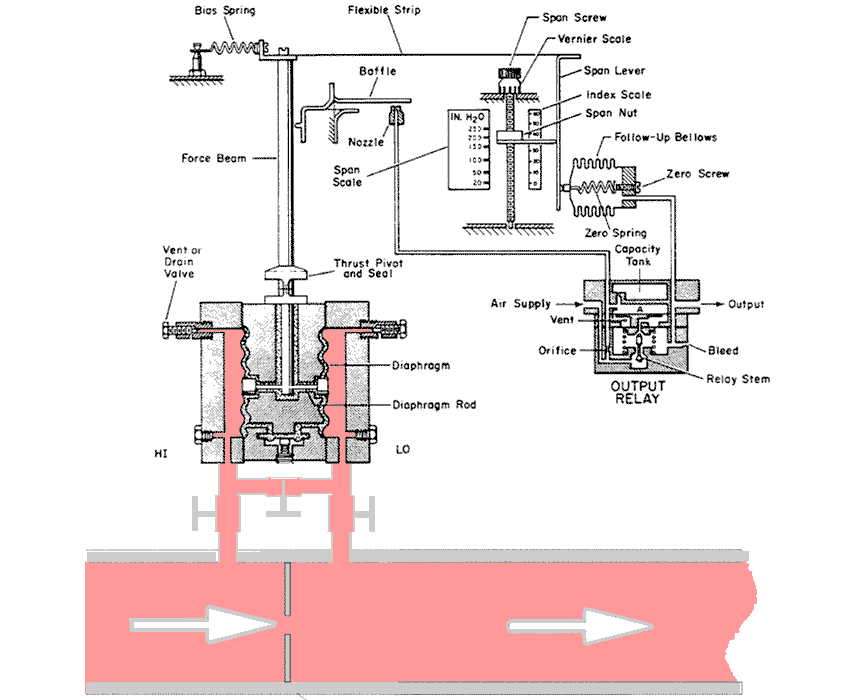Differential Pressure Transmitter setup to measure flowrate.
A d/P transmitter measures differential pressure. In the pipe,
the pressure drop across the restriction will be sensed and
not the 100psi static pressure.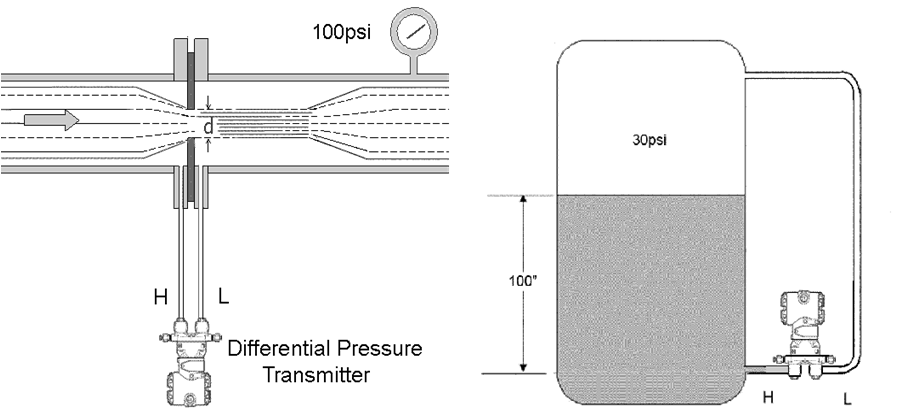In the tank the transmitter senses pressure equivalent of the liquid height.
The 30psi static pressure is not sensed since it is applied to both sides.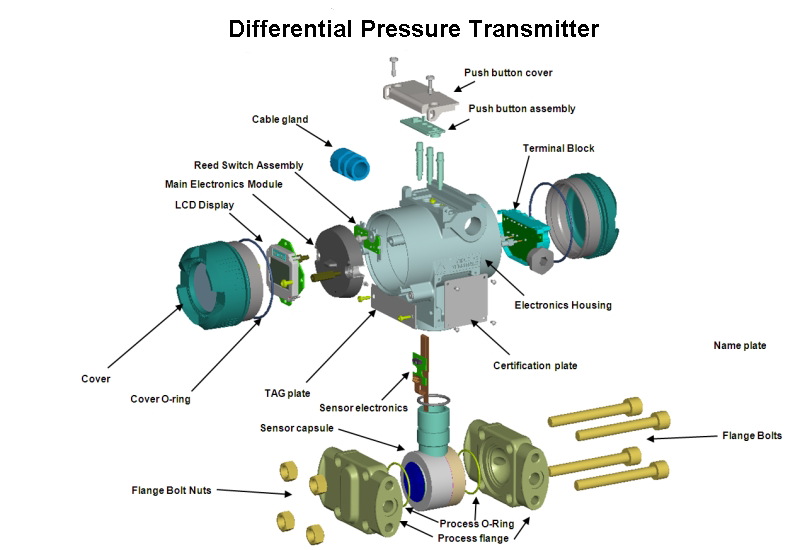To calibrate a pressure instrument we need a very accurate reference pressure Dead-weight pressure calibrator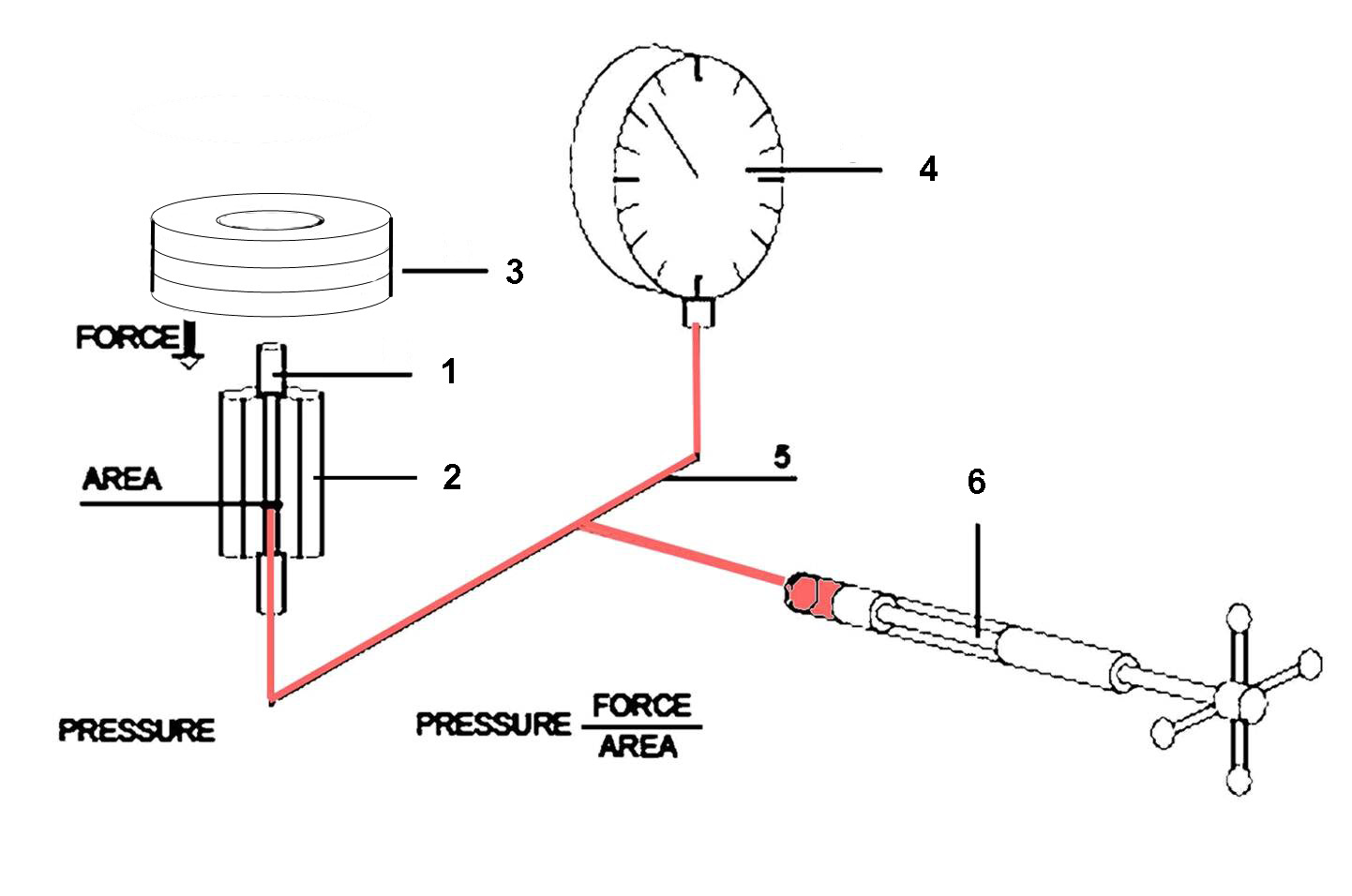The principle of a deadweight tester is very simple. A deadweight tester consists of a piston/cylinder combination and a set of weights. The area of the piston and the mass of the weights have been accurately determined. The calibration fluid 5 is pressurized by means of a ram screw 6 to the point where the piston 1, including the weights 3, starts to float, whereby the forces are balanced. Since From Newton’s law force (F) is equal to mass (m) times acceleration (g). Pressure (P) is equal to force divided by area (A). Example: The area of the piston is one square inch, the set of weights is 10 pounds, therefore the pressure generated is

P = F = 10 lbs     = 10 psi

A = 1"

The pressure gauge should also read 10 pounds per square inch.

High stability

High repeatability